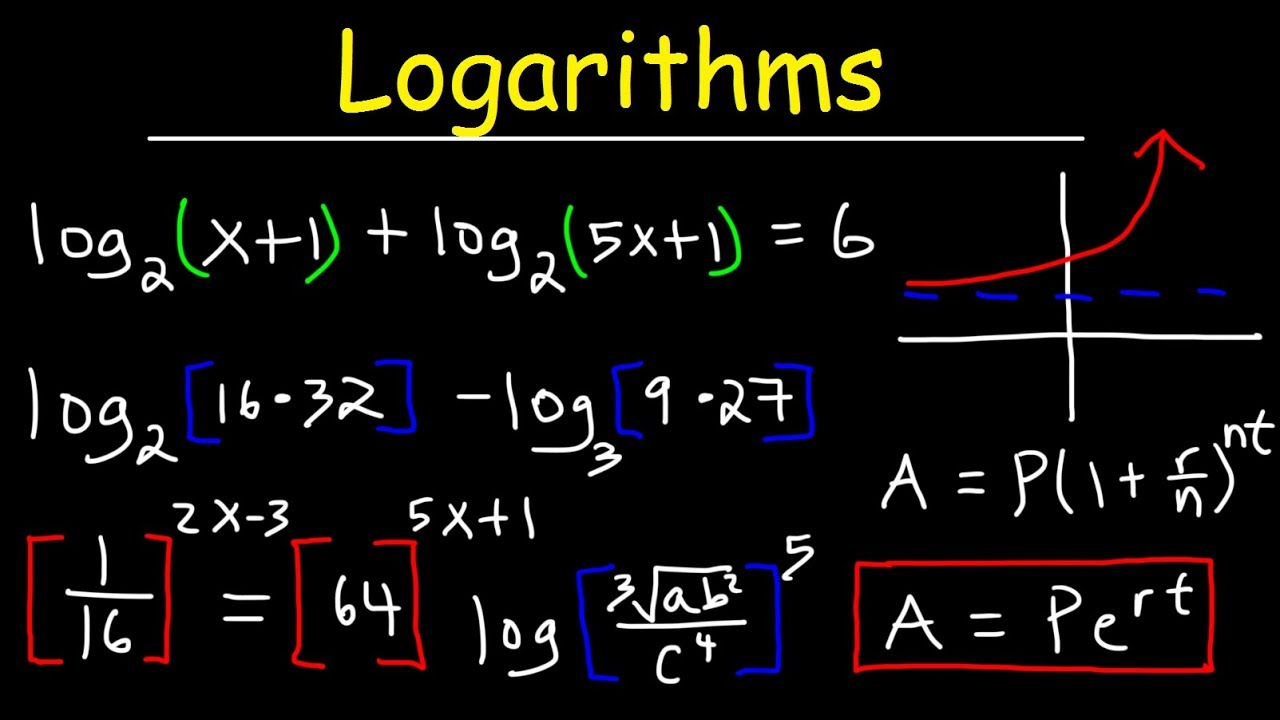# My Poll Ux - Internet of things

## Thursday, October 8, 2020

The index or the power to which a number must be raised in order to obtain some other number is called the logarithm. It is abbreviated as ‘log’. It was introduced by John Napier. It is the inverse function of exponentiation. That means the log of a given number x is the exponent to which another fixed number, the base b, must be raised, to produce that number x. It counts the number of occurrences of the same factor in repeated multiplication. For example, 100 = 10×10 = 102. Here log 100 = 2. The power is 2 and the base is 10. The log of x to base b is denoted as logb x.Exponentiation allows any positive real number as a base to be raised to any real power, which will always produce a positive result, so logb(x) for any two positive real numbers b and x, where b ≠1, is always a unique real number y. The relation between exponentiation and logarithm is:

logb(x) = y if by = x and x >0, b>0 and b ≠ 1.

For example, log2 32 = 5, since 25 = 32.

Also, log2 64 = 6, since 26 = 64.

Base 10 log is known as the common log. The natural log has a base e. I.e b ≈ 2.718. ‘e’ is called Napier’s base. The integral part of the log is called the characteristic and the fractional part or the decimal part is called the mantissa. i.e., log N = Integer + Fractional or decimal part (+ve). The mantissa is always kept positive.

## Properties of Characteristic and Mantissa

When the characteristics of log N is n, the number of digits in N is (n+1) (Here, N > 1).

When the characteristics of log N is -n, there exists (n-1) number of zeroes after decimal part of N (here, ) < N < 1).

When N > 1, the characteristic of log N will be on less than the number of digits in an integral part of N.

When 0 < N < 1, the characteristic of log N is -ve, and numerically it is one greater than the number of zeroes just after the decimal part in N.

### Laws of Logarithm

1. log A + log B = log AB. This law says how to add two logs together.

2. log A - log B = log(A/B). Subtraction of log B from log A gives log(A/B).

3. Log An = n log A.

4. log 1 = 0

5. loga a = 1.

### Probability JEE Main Previous Year Questions

Probability is an important topic for the JEE exam. Students are recommended to revise and learn probability JEE Main previous year questions with solutions. These solutions will help students to have knowledge of the type of questions asked for the JEE Main exam. Students can easily download these solutions in PDF format from the website.

All the solutions are given in a step by step manner so that students can easily understand the problems. Practising previous year question papers help students to improve their problem-solving skills and accuracy. This helps students to be stress-free and confident during the exam.

#### Post a Comment

Note: Only a member of this blog may post a comment.